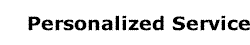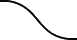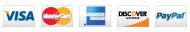Custom Extension Spring Manufacturer### MS24585-Custom Spring ManufacturerToll Free: 1 (888) 322-9974

# Custom Extension Spring Manufacturer

Extension Springs are close-coiled helical springs designed to resist an axial pulling force. Extension Springs or tension springs stretch from free length to a longer extended length to store energy, and offer resistance or provide a restoring force to a pulling force between two points.

Most extension springs are manufactured from round wire and are close wound with initial tension which offers a small deflection load for secure installation. You can design an extension spring with open wound coils when zero initial tension is required or when the spring needs to perform as an extension and a compression spring for the same application. Optimum Spring manufactures custom extension springs in different lengths and degrees of tension to suit your specifications.## Configuration

Common extension spring ends we manufacture are:

• Hook: crossover center, extended, full round, machine
• Loops: side, single full, double full

## Physical Parameters

• d (Wire Diameter): Wire diameter for the spring material.
• De (External/Outside Diameter): Calculated by adding the internal diameter plus two times the wire diameter of a spring.
• H (Hole): Minimum diameter of the hole in which a spring works.
• L0 (Free Length): Overall length is specified between the inside of the spring loops.
• Number of Coils: This is a total number of coils in a spring; in this case it is equal to six.

## Performance Factors

• P (Pitch): Average distance between two subsequent active coils of a spring.
• Ln (Maximum Loaded length): Maximum acceptable extension length for a spring
• Fn (Maximum Force): Maximum acceptable force which can operate on a spring.
• R (Spring Rate/Stiffness): the change in load per unit deflection in pounds per inch (lb/in) or Newtons per millimeter (N/mm).
• L1 & F1 (Length at Force F): Force F1 at Length L1 can be calculated from equation:
F1 = Fn - R(Ln-L1).
Equation derived from the previous for calculating L1 = Ln - (Fn-F1)/R.
• Ends Shape: Loops or hooks.
• Winding Direction: Helix direction is either right hand or left hand. Handedness is important in very few applications.

## Wire Diameter

We manufacture extension springs with a wire diameter of 0.004" up to 0.120" (0.1mm to 3.0mm)

## Wire Material

Music wire , stainless steel, and hard drawn are the most common materials we use to manufacture extension springs. Other materials we use and their properties are listed in the Materials table.

## Wire Selection

Round wire is most commonly used for extension springs because it is most adaptable to standard coiler tooling. We can manufacture extension springs with square, rectangular and special wire sections.

An optimum extension spring depends on:
• Operating Environment
• Space
• Energy
• Service Life /Fatigue

## Design Decisions

Most failures occur in the extension spring ends. Consider having a smooth, gradual and seamless path of wire from the body of the spring to its ends to maximize the life of the spring.

• High Temperature Environment: Consider having extra-tight coils when designing extension springs because heat may reduce the spring life span.
• Stress Level: Determined by the dimensional limits along with the load and deflection requirements.
In the body of an extension spring the wire is stressed in torsion. You must include the stress from initial tension to calculate the total stress. When estimating the maximum tolerable stress, the stresses in the spring ends must also be considered.
• Plastic Deformation: This non-reversible change of shape in response to an applied force may deform the spring and it may not return to its initial condition with all coils closed. For that reason, there should be some mechanical limit on how far the spring can be extended.
• Initial Tension: Equal to the minimum force required to separate adjacent coils. The amount of this build-in load is a related to the material type, material size, and the spring index which is determined by the mean coil diameter divided by the wire diameter.
Initial tension is measured by extending the coil to a length (L1) sufficient to open coils and measure load (P1). Extend spring to length (L2) such that second deflection equals first deflection and again measure load (P2). The amount of Initial Tension is equal to two times the load achieved at (L1) minus the load at (L2). The formula for Initial Tension (Pi) = 2P1 - P2.
If an extension spring with no initial tension is needed for your application, you can design the spring with space between the coils.
• Spring Rate: Lbs. of load per inch of deflection. The rate is frequently determined by two load requirements at two working lengths. It is measured by extending the coil to a length (L1) such that coil separation occurs and measure the load (P1). Extend the spring further to a second length (L2) and again measure the load (P2). Calculate the rate by dividing the load difference by the length difference in: R =(P2 - P1)/(L2 - L1).
The rate is most linear between 20% and 80% of the available deflection.
• Load: The load of an extension spring is calculated by multiplying the Spring Rate times the deflection and adding the Initial Tension. It is usual to assign the load at an extended length, as opposed to a load at a specific deflection.
• Maximum Deflection: Determined by subtracting the Initial Tension from the Maximum Load. This result then divided by the Spring Rate.
The formula for Maximum Deflection is (Maximum Load – (Pi)) ÷ R

## Production

We offer fast turnaround on small, medium and large runs. In addition, we welcome any extension spring projects which require limited quantities. Our design specialists offer you specific advice and design review during the prototyping process.

## Applications

Our extension springs go into many applications for diverse industries that require high-quality coil springs. See a list of Industries we serve.

Call
1 (888) 322-9974

How Can We Help You?

Name Email MessagePhone: +1 (904) 567-5999 • solutions@OptimumSpring.com 150 Hilden Rd • Suite 316 • Ponte Vedra, FL 32081-8405 U.S.A